# Ex 12.2 NCERT Solutions- Areas Related to Circles Class 10 Notes | EduRev

## Class 10 : Ex 12.2 NCERT Solutions- Areas Related to Circles Class 10 Notes | EduRev

The document Ex 12.2 NCERT Solutions- Areas Related to Circles Class 10 Notes | EduRev is a part of the Class 10 Course Mathematics (Maths) Class 10.
All you need of Class 10 at this link: Class 10

NCERT TEXTBOOK QUESTIONS SOLVED
Page No. 230
(EXERCISE 12.2)

Use π = 22/7 (unless stated otherwise)
Q 1. Find the area of a sector of a circle with radius 6 cm if angle of the sector is 60°.
Sol. Here,
r = 6 cm
θ = 60°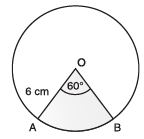∴ Using, the Area of a sector =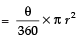We have,
Area of the sector with r  = 6 cm and θ = 60°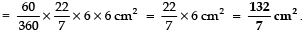Q 2. Find the area of a quadrant of a circle whose circumference is 22 cm.
Sol. Let radius of the circle = r
∴ 2πr = 22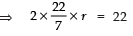⇒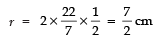Here θ = 90°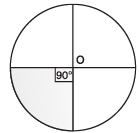∴ Area of the quadrant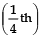of the circle,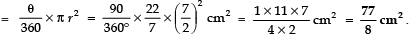Q 3. The length of the minute hand of a clock is 14 cm. Find the area swept by the minute hand in 5 minutes.
Sol. [Length of minute hand] = [radius of the circle]
⇒ r = 14 cm
∵ Angle swept by the minute hand in 60 minutes = 360°
∴ Angle swept by the minute hand in 5 minutes =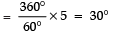Now, area of the sector with r = 14 cm and θ = 30°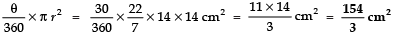Thus, the required area swept by the minute hand by 5 minutes = 154/3 cm2.

Q 4. A chord of a circle of radius 10 cm subtends a right angle at the centre. Find the area of the corresponding (i) minor segment (ii) major sector. (Use π = 3.14)
Sol. Given, radius of the circle = 10 cm
Angle subtend by chord at centre = 90° ...(i)
(i) Area of the minor segment = (Area of the sector OAB) - (Area of ΔAOB formed with radius and chord)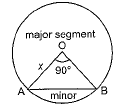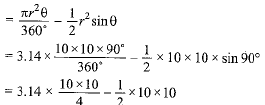= 3.14 x 25 - 50 = 78.5 - 50 = 28.5 cm2
(ii) Area of the major segment = Area of the circle - Area of the minor segment
= πr2 - 28.5 = 3.14 x 10 x 10 - 28.5
= 314 - 28.5 = 285.5 cm2

Q 5. In a circle of radius 21 cm, an arc subtends an angle of 60° at the centre. Find:
(i) the length of the arc
(ii) area of the sector formed by the arc
(iii) area of the segment formed by the corresponding

Sol.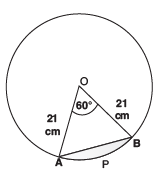Here, radius = 21 cm and θ = 60°
(i) Circumference of the circle = 2πr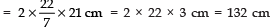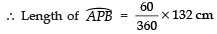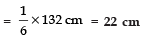(ii) Area of the sector with sector angle 60°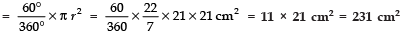(iii)  Area of the segment formed by the corresponding chord - area of the sector - area of the Δ formed between chord and radius of the circle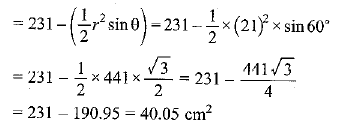Q 6. A chord of a circle of radius 15 cm subtends an angle of 60° at the centre. Find the areas of the corresponding minor and major segments of the circle.  (Use π = 3.14 and √3 = 1.73)
Sol.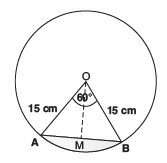Radius of the circle = 15 cm
Central angle subtends by chord = 600
Area of sector =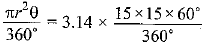= 117.75 cm2
Area of the triangle formed by radii and chord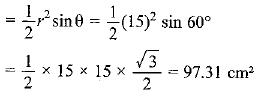Area of the minor segment = Area of the sector -Area of the triangle formed by radii and chord
= 117.75-97.31 =20.44 cm2
Area of the circle = πr2
= 3.14 x 15 x 15 = 706.5 cm2
Area of the major segment = Area of the circle - Area of the minor segment
= 706.5 - 20.44 = 686.06 cm2

Q 7. A chord of a circle of radius 12 cm subtends an angle of 120° at the centre. Find the area of the corresponding segment of the circle. (Use π = 3.14 and √3 = 1.73)
Sol. Here, θ = 120° and r = 12 cm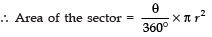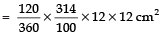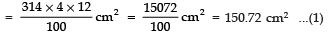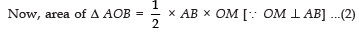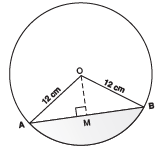In Δ OAB, ∠O = 120°
⇒∠A + ∠B = 180° − 120 = 60°
∵ OB = OA = 12 cm ⇒∠A = ∠B = 30°
So,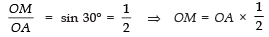⇒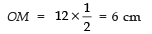In right Δ AMO, 122 − 62 = AM2
⇒ 144 − 36 = AM2
⇒ 108 = AM2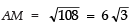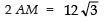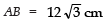Now, from (2),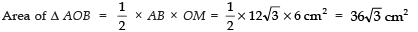= 36 × 1.73 cm2 = 62.28 cm2     ...(3)
From (1) and (3)
Area of the minor segment = [Area of minor segment] − [Area of Δ AOB]
= [150.72 cm2] − [62.28 cm2] = 88.44 cm2.

Q 8. A horse is tied to a peg at one corner of a square shaped grass field of side 15 m by means of a 5 m long rope (see figure). Find:
(i) the area of that part of the field in which the horse can graze.
(ii) the increase in the grazing area if the rope were 10 m long instead of 5 m. (Use π = 3.14)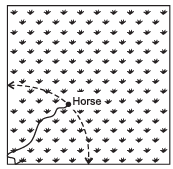Sol. Here, Length of the rope = 5 m
∴ Radius of the circular region grazed by the horse = 5 m
(i) Area of the circular portion grazed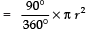[∵ θ = 90° for a square field.]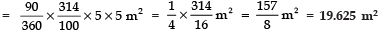(ii) When length of the rope is increased to 10 m,
∴ r = 10 m
⇒ Area of the circular region where θ = 90°.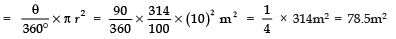∴ Increase in the grazing area = 78.5 − 19.625 m2 = 58.875 m2.

Q 9. A brooch is made with silver wire in the form of a circle with diameter 35 mm. The wire is also used in making 5 diameters which divide the circle into 10 equal sectors as shown in Fig. Find: (i) the total length of the silver wire required. (ii) the area of each sector of the brooch.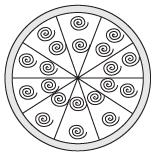Sol. Diameter of the circle = 35 mm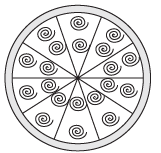∴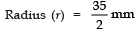(i) Circumference =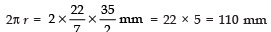Length of 1 piece of wire used to make diameter to divide the circle into 10 equal sectors = 35 mm
∴ Length of 5 pieces = 5 × 35 = 175 mm
∴ Total length of the silver wire = 110 + 175 mm = 285 mm
(ii) Since the circle is divided into 10 equal sectors,
∴ Sector angle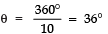⇒ Area of each sector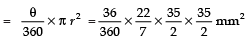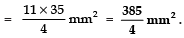Page No. 231
Q 10. An umbrella has 8 ribs which are equally spaced (see figure). Assuming umbrella to be a flat circle of radius 45 cm, find the area between the two consecutive ribs of the umbrella.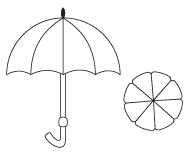Sol. Here, radius (r) = 45 cm
Since circle is divided in 8 equal parts,
∴ Sector angle corresponding to each part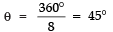⇒ Area of a sector (part)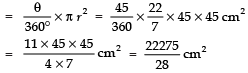∴ The required area between the two ribs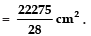Q 11. A car has two wipers which do not overlap. Each wiper has a blade of length 25 cm sweeping through an angle of 115°. Find the total area cleaned at each sweep of the blades.
Sol. Here, radius (r) = 25 cm
Sector angle (θ) = 115°
∴ Area cleaned by each sweep of the blades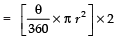[∵ Each sweep will have to and fro movement]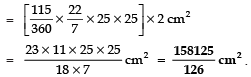Q 12. To warn ships for underwater rocks, a lighthouse spreads a red coloured light over a sector of angle 80° to a distance of 16.5 km. Find the area of the sea over which the ships are warned. (Use π = 3.14)
Sol. Here, Radius (r) = 16.5 km
Sector angle (θ) = 80°
∴ Area of the sea surface over which the ships are warned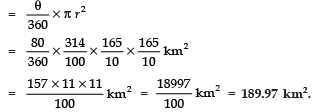Q 13. A round table cover has six equal designs as shown in Fig. If the radius of the cover is 28 cm, find the cost of making the designs at the rate of Rs 0.35 per cm2. (Use √3 = 1.7)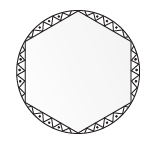Sol. Here,    r = 28 cm
Since, the circle is divided into six equal sectors.
∴ Sector angle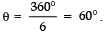∴ Area of the sector with θ = 60° and r = 28 cm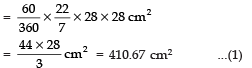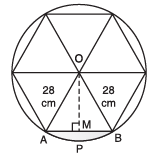Now, area of 1 design
= Area of segment APB
= Area of sector − Area of ΔAOB  ...(2)
In ΔAOB, ∠AOB = 60°, OA = OB = 28 cm
∴   ∠OAB = 60° and ∠OBA = 60°
⇒ ΔAOB is an equilateral triangle.
⇒ AB = AO = BO
⇒ AB = 28 cm
Draw OM ⊥ AB
∴ In right ΔAOM, we have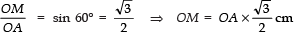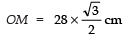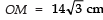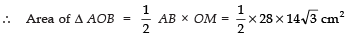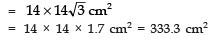...(3)
Now, from (1), (2) and (3), we have:
Area of segment APQ = 410.67 cm2 − 333.2 cm2 = 77.47 cm2
⇒ Area of 1 design = 77.47 cm2
∴ Area of the 6 equal designs = 6 × (77.47) cm2
= 464.82 cm2
Cost of making the design at the rate of Rs 0.35 per cm2,
= Rs 0.35 × 464.82
= Rs 162.68.

Q 14. Tick the correct answer in the following: Area of a sector of angle p (in degrees) of a circle with radius R is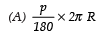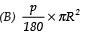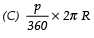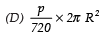Angle of sector (θ)= p°
∴ Area of the sector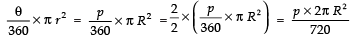Thus, the option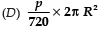is correct.

Offer running on EduRev: Apply code STAYHOME200 to get INR 200 off on our premium plan EduRev Infinity!

## Mathematics (Maths) Class 10

178 videos|281 docs|103 tests

,

,

,

,

,

,

,

,

,

,

,

,

,

,

,

,

,

,

,

,

,

;